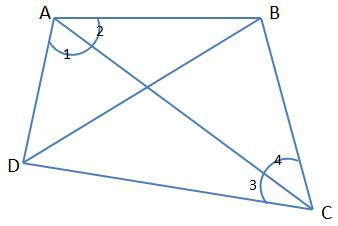Any closed figure in plane, bounded by four line segments is called a Quadrilateral. In the given quadrilateral$ABCD$:i) Four vertices are$A, \ B, \ C, \ and\ D$

ii) Four line segments are$AB,\ BC,\ CD,\ DA$

iii) Four angles are$\angle A,\ \angle B, \ \angle C \ and \ \angle D$

iv) Two diagonals are$AC \ and\ BD$

Let’s also learn the nomenclature related to quadrilaterals:

1) Adjacent Sides: Two sides of a quadrilateral having a common vertex. Example$AD \ and\ DC$ are adjacent sides with D as the common vertex. Similarly,$AB \ and\ BC$ are adjacent sides with common vertex B.

2) Opposite Sides: Two sides of a quadrilateral that doesn’t share a common vertex. Example:$AB \ and\ DC$ are opposite side. Similarly,$AD \ and\ BC$ are opposite sides. You will also see that they don’t share any common vertex.

3) Adjacent Angles: Two angles of a quadrilateral having a common arm are called adjacent angles Example: In the above figure$\angle A \ and\ \angle B$ are adjacent and they share$AB$ as the common arm. Similarly,$\angle B \ and\ \angle C$ are adjacent angles and they share$BC$ as the common arm.

4) Opposite Angles: Angles in a quadrilateral that doesn’t share any common arm are called opposite angles. Example: In the above figure,$\angle A \ and\ \angle C$ are opposite angles. Similarly,$\angle D \ and\ \angle B$ are opposite angles.

Important Theorem:

Sum of the interior angles$= (n-2) \times 180=(2n-4) \times 90^{\circ}$

In a quadrilateral,$n=4$

Substituting in the above formula, we get that the sum of the internal angles is$360^{\circ}$

Another way of proving this is as follows:In$\Delta ABC \colon \angle 2 + \angle 4 + \angle B = 180^{\circ} ...i)$

Similarly, in$\Delta ADC: \angle 1 + \angle 3 + \angle D = 180^{\circ} ...ii)$

Adding i) and ii) we get$(\angle 1 + \angle 2) + (\angle 4 + \angle 3) + \angle D + \angle B = 360^{\circ}$

Or$\angle A+\angle B+\angle C+\angle D=360^{\circ}$